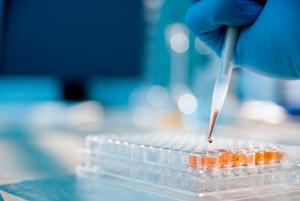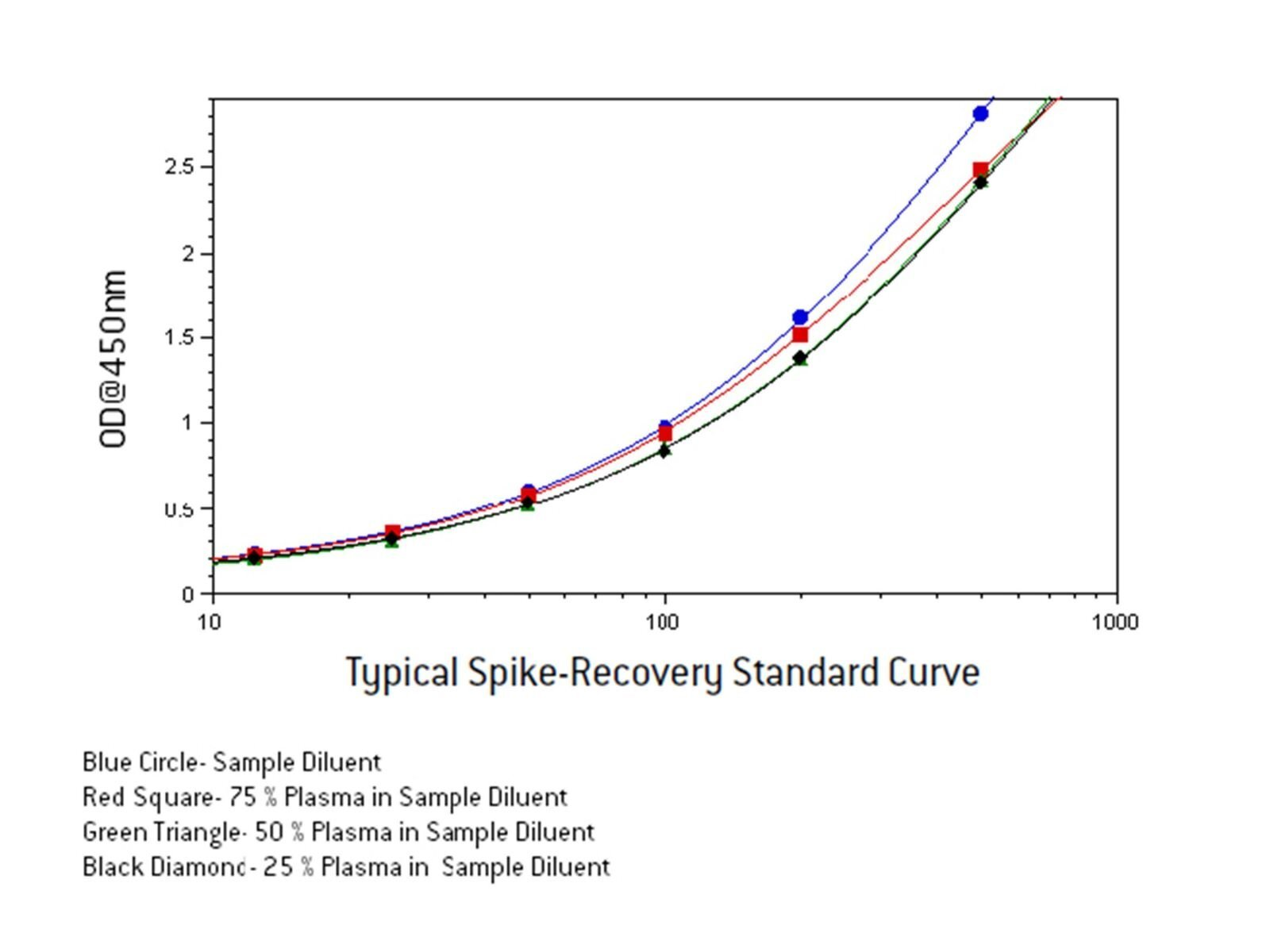# General Spike and Recovery Protocol For ELISA

Hero Region
ImageBackground

The Spike and Recovery method is an important technique for analyzing and accessing the accuracy of ELISA and other analytical methods for particular sample types. It is used to determine whether analyte detection can be affected by the difference between diluent used for preparation and the experimental sample matrix.

To perform a spike and recovery experiment, a known amount of analyte is added to a matrix which does not contain endogenous analyte and the same known amount of analyte is added to a regular diluent. The concentration of the added analyte in the both cases is determined from standard curves prepared in the respective matrices. The concentrations denote the spike recovered in the two cases. If the recovery differs, then components in the sample matrix are causing the difference, and adjustments must be made to the method to minimize the discrepancy. Here, we provide a general spike and recovery protocol for the determination of concentration of an analyte (Human Interferon Alpha) in serum.

### Determination of concentration of analyte (Human IFN-α) in serum samples using kit 41110-1 Materials: Solution 1: Neat Human Serum without endogenous amounts of interferon-alpha Solution 2: Human Serum samples with unknown amounts of interferon-alpha 41110-1/-2 VeriKine Interferon Alpha Multi-Subtype Serum ELISA kits Note: Spike is same as analyte   Prepare a 2 X standard curve (i.e. 25-1000 pg/ml) in Solution 1.Dilute the 2 X standard curve 1:1 in sample diluent to give a final 1 X Standard curve in 50% Solution 1 (50% serum). Prepare a 1 X Standard curve in sample diluent. Preparation of samples: Known spike (diluted in Solution 1) + sample diluent in 1:1 ratio. (Note: the known spike will be diluted by half. Ensure that the reduced concentration of the spike lies on the std. curve). Known spike (diluted in sample diluent) + serum sample with an unknown amount of analyte in a 1:1 ratio. (Note: It is recommended to add a spike with concentration of that added in sample A). Sample diluent + Serum sample used to prepare sample B, in 1:1 ratio. Known spike used in sample B + sample diluent in a 1:1 ratio Load the standard curves (prepared in steps 1-2) and samples A, B, and C onto the plate in duplicates and run the ELISA as per the protocol provided in the kit. Read the plate at 450 nm and fit the data points of the standard curve using a 4-parameter non-linear fit. Back-calculate the concentration of the samples based on their mean OD values using the standard curves. (Note: Ensure that the concentrations of the samples are back-calculated from standard curves prepared in the same dilution of serum, i.e. concentrations of samples A, B and C must be back-calculated from std. curve prepared in step 1. Concentration of sample D must be back-calculated from standard curve prepared in step 2). Spike and Recovery test: The recovery of sample A = 2 x back-calculated value of sample A x 100/known value of spike. The recovery must be between 90-110% to qualify solution 1. The recovery of sample D= 2 x back-calculated value of sample D x 100/known value of spike. The recovery must be between 90-110% to qualify solution 1. This value must be within +/- 10 % of value from 7-A The following criteria is used to determine whether there are any interfering components in the unknown serum sample: Back calculated concentration of sample B = Back-calculated concentration of sample A + Back-calculated concentration of sample C. The recommended acceptable deviation is +/- 10%. If both criteria from step 7 are met; the concentration of Interferon-Alpha in unknown sample A = 2 (dilution factor) x back-calculated concentration of sample A.We strongly recommend that every time serum samples are tested: A standard curve is prepared in 50 % normal serum which has been previously qualified to be free of endogenous concentrations of analyte (IFN alpha) according to the criteria in steps 7.1 and 7.2 Serum samples are diluted to 50% in sample diluent. Sample A is prepared with at least one concentration of a known spike. Whether or not a spike and recovery test is performed for every sample in cases where there are several unknown samples to test is at the discretion of the operator.

Image## Related Article

General Protocol For Human IFN-Alpha Cytopathic Effect (CPE) Assay